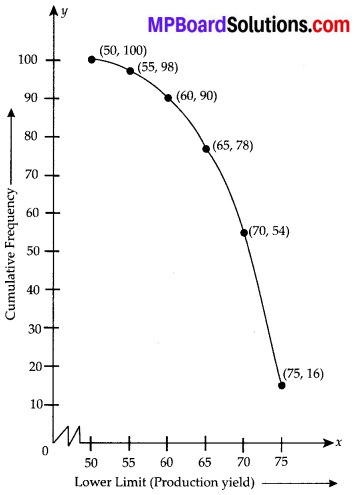In this article, we will share MP Board Class 10th Maths Book Solutions Chapter 14 Statistics Ex 14.4 Pdf, These solutions are solved subject experts from the latest edition books.

## MP Board Class 10th Maths Solutions Chapter 14 Statistics Ex 14.4

Question 1.
The following distribution gives the daily income of 50 workers of a factory.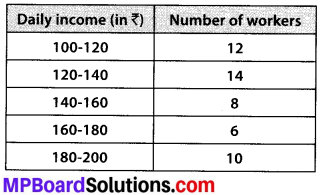Convert the distribution above to a less than type cumulative frequency distribution, and draw its ogive.
Solution:
We have the cumulative frequency distribution as follows: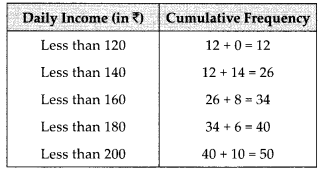Now, we plot the points corresponding to the ordered pair (120, 12), (140, 26), (160, 34), (180, 40) and (200, 50) on a graph paper and join them by a free hand to get a smooth curve as shown below: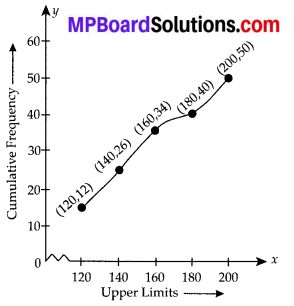The curve so obtained is called the less than ogive.Question 2.
During the medical check-up of 35 students of a class, their weights were recorded as follows: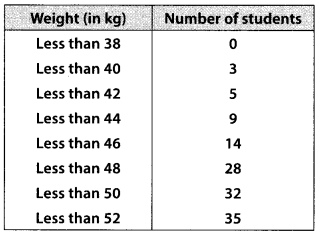Draw a less than type ogive for the given data. Hence obtain the median weight from the graph and verify the result by using the formula.
Solution:
Here, the values 38, 40, 42, 44, 46, 48, 50 and 52 are the upper limits of the respective class-intervals.
We plot the points (ordered pairs) (38, 0), (40, 3), (42, 5), (44, 9), (46,14), (48, 28), (50, 32) and (52, 35) on a graph paper and join them by a free hand to get a smooth curve.
The curve so obtained is the less than type ogive.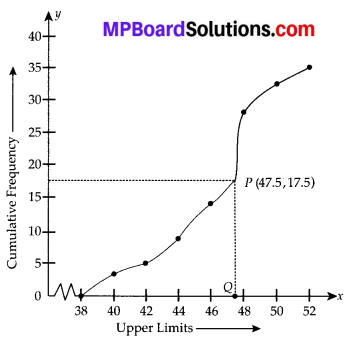∵ N = 35
∴ $$\frac{N}{2}=\frac{35}{2}$$ = 17.5
The point 17.5 is on y-axis.
From this point (i.e., from 17.5) we draw a line parallel to the x-axis which cuts the curve at P. From this point P, draw a perpendicular to the x-axis, meeting the x-axis at Question The point Q represents the median of the data which is 47.5.
Verification:
To verify the result, let us make the following table in order to find median using the formula :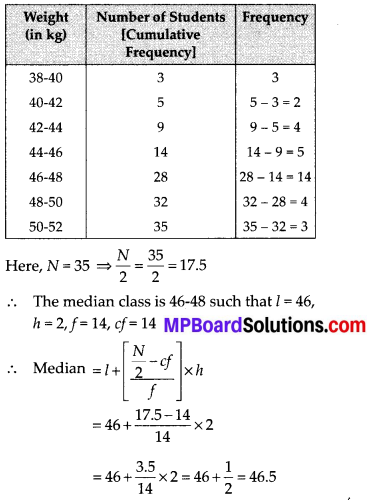Thus, the median = 46.5 kg is approximatelyQuestion 3.
The following table gives production yield per hectare of wheat of 100 farms of a village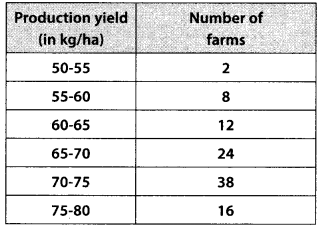Change the distribution to a more than type distribution, and draw its ogive.
Solution:
For more than type distribution, we have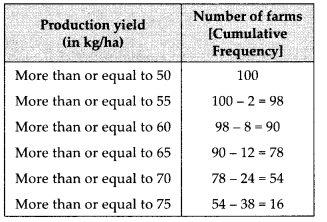Now, we plot the points (50, 100), (55, 98), (60, 90), (65, 78), (70, 54) and (75, 16) and join the points with a free hand to get a smooth curve.
The curve so obtained is the ‘more than type ogive’.